# Important Questions for Class 12 Maths Chapter 3 - Matrices

Important Questions for Class 12 Maths Chapter 3 matrices are provided here. Students can practise these questions for the board examination to score well. The important questions are taken as per the syllabus of CBSE board. Practice these important problems, which are asked in the previous year board examination. Also, get the important questions for class 12 Maths here at BYJU’S.

In class 12 maths, chapter 3 contains concepts such as matrices and its order, equality, transpose of a matrix, zero and identity matrix, operations and properties of matrices, elementary row and column operation, invertible matrices, symmetric and skew-symmetric matrices with more examples.

Also, check:

## Class 12 Chapter 3 Matrices Important Questions With Solutions

Practice the important questions for class 12 chapter 3 matrices to score good marks in the board examinations. Be thorough with these problems, as these problems are taken from the previous year Maths examination papers.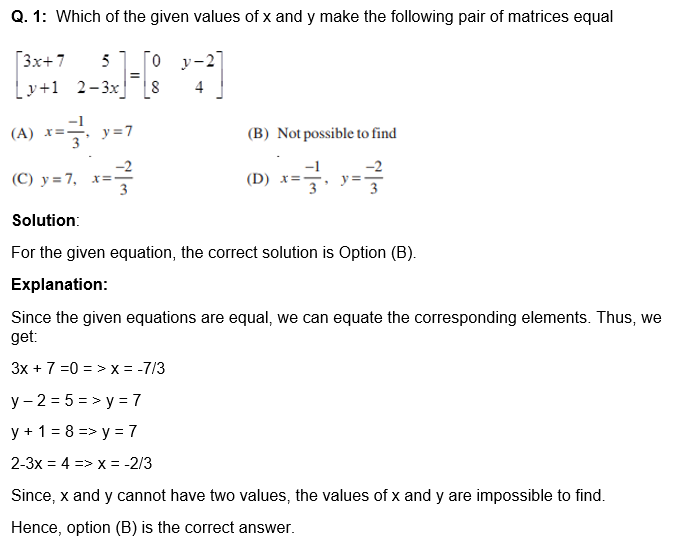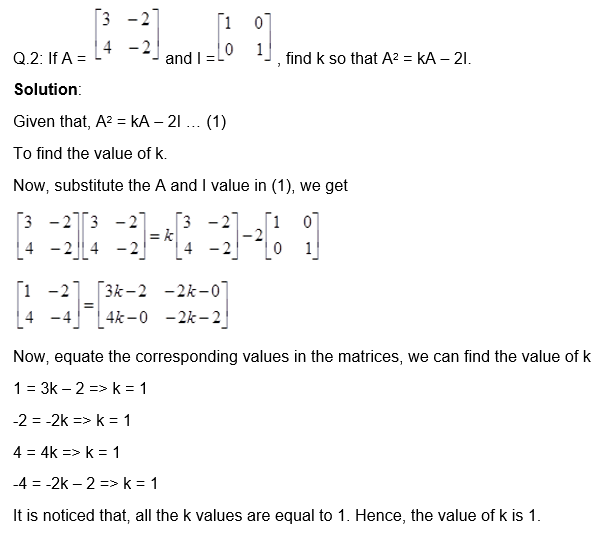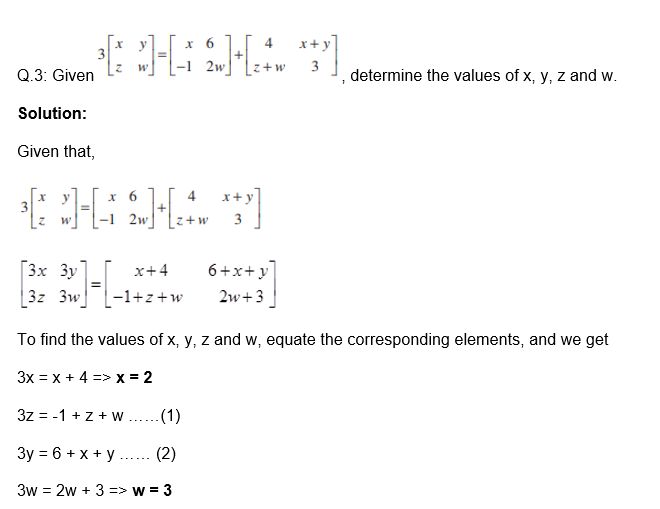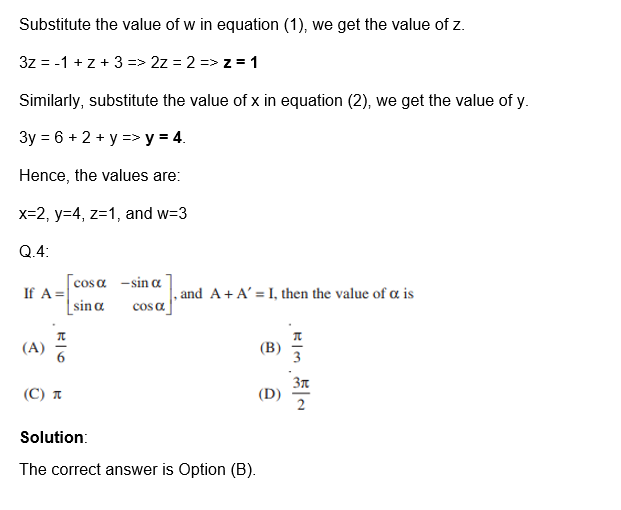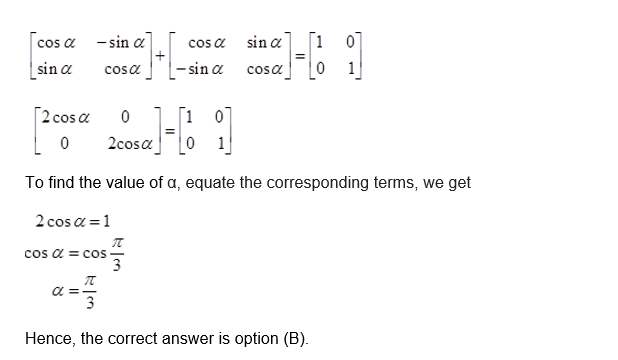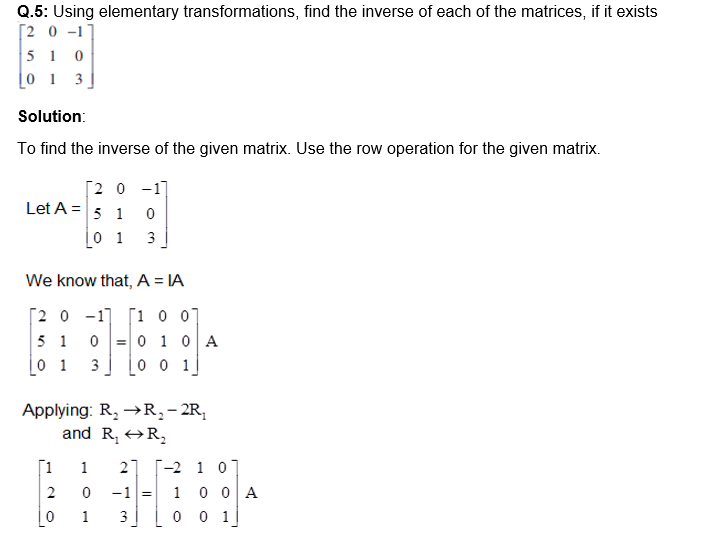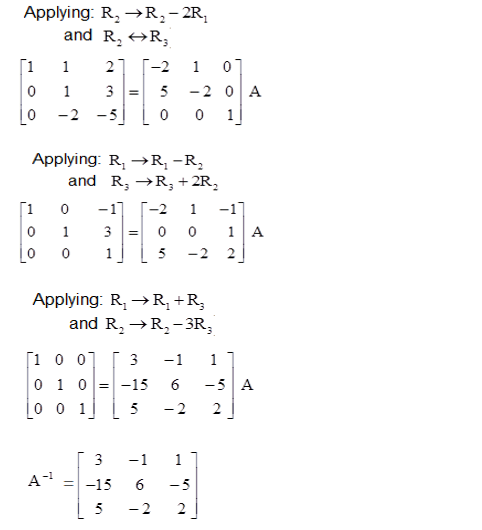### Practice Problems for Class 12 Chapter 3 Matrices

Solve the below-given important problems in chapter 3 Matrices.

1. Determine the inverse of each of the matrices, if it exists. $$\begin{bmatrix} 2 & -3 & 3\\ 2 & 2 & 3 \\ 3& -2 & -2 \end{bmatrix}$$
2. Express the given matrix as the sum of the symmetric and the skew-symmetric matrix. $$\begin{bmatrix} 3 & 5 \\ 1 & -1 \end{bmatrix}$$
3. If A = $$\begin{bmatrix} 3 & -4\\ 1 & -1 \end{bmatrix}$$, then prove that An = $$\begin{bmatrix} 1+2n & -4n \\ n & 1-2n \end{bmatrix}$$, where n is an any positive integer.
4.  Determine the values of the variables such as a, b, c and d from the given equation: $$\begin{bmatrix} a -b & 2a+c \\ 2a-b & 3c+d \end{bmatrix} = \begin{bmatrix} -1 & 5 \\ 0 & 13 \end{bmatrix}$$

For more important questions for class 12 maths, download BYJU’S – The Learning App and practice all the important problems in Maths.

#### 1 Comment

1. yeah, good ,this zone, seems so comfortable.